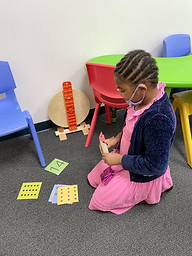## Ms. Caitlyn

### Target 1​

###### Lesson Type:

New

Number Operation

:

Number System

Skip count by tens up to 100.

###### 1:

Connect skip counting to repeated addition and subtraction.

###### 2:

Discover counting patterns through skip counting by tens from zero to 100.

###### 3:

Connect skip counting by tens to place-value in the tens’ place.

1st

###### Vocabulary:

Skip Count, Hundreds Chart

Activities:

• Students used a hundreds' chart to skip count by 10s, then were challenged to skip count by 2s and 5s.
• Students recognized addition and subtraction patterns in skip counting.### Home Exploration

###### Guiding Questions:## Absent Students:

### Target 2

:

###### 1:

Count objects to determine how many there are.

###### 2:

Recognize the quantities zero to ten, without having to count.

###### 3:

Develop strategies of grouping quantities to count larger quantities faster (i.e. base-ten blocks).

Kindergarden

###### Vocabulary:

Activities:

• Students matched a number of dots to the written number.
• Students drew number cards and created them with base-ten blocks.### Home Exploration

###### Guiding Questions:### Target 3

:

###### 1:

Write numbers correctly.

###### 2:

Recognize a number without having to see its position in the count sequence.

Kindergarden

###### Vocabulary:

Activities:

• Students were given a quantity of base-ten blocks and wrote the number on their white boards.
• Students practiced writing numbers in the proper direction (i.e.: fixing backwards 7s and 5s).
• Students practiced writing the numbers in the tens (12, 13, 14, etc.), noting that the 1 is in the tens place.
• Students played Number Bingo, where the teacher gave them a set of base ten blocks and they had to find the number on their card.### Home Exploration